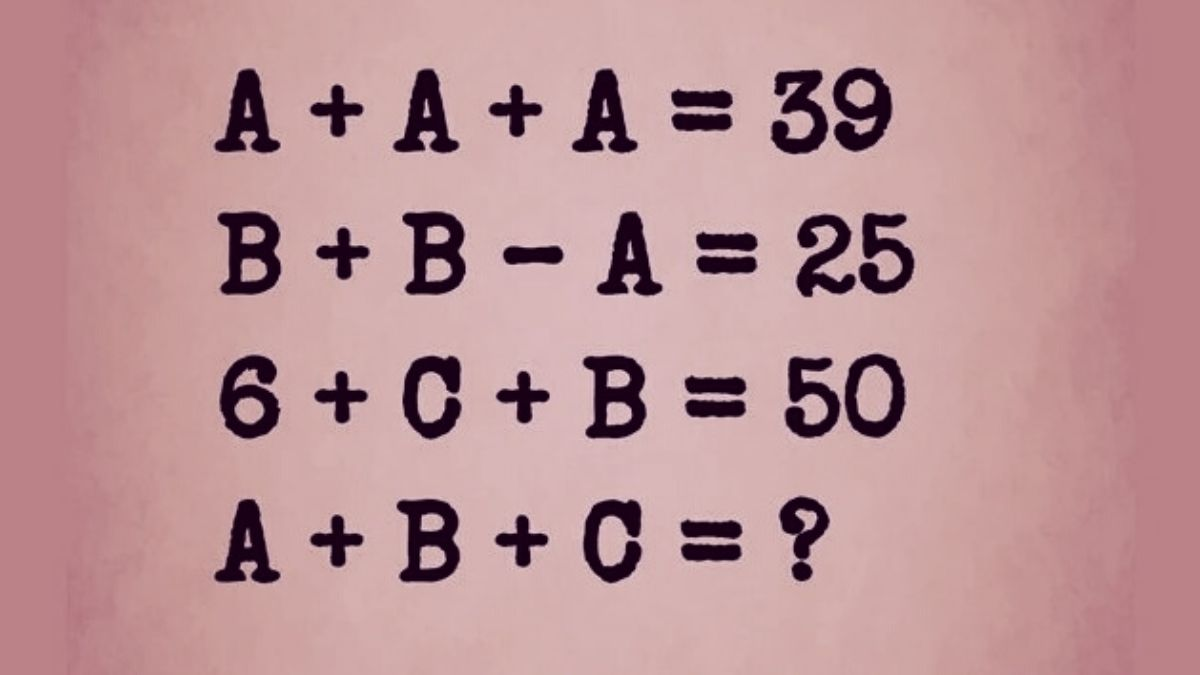# Math Riddles: Can you solve this math riddle in 15 Seconds?

Math Riddles are intriguing puzzles in which you must solve the riddle to determine the value of the item requested in the riddle. Test your math skills by attempting this math riddle now.Math Riddles - Find the value of A+B+C

Math Riddles: Basic mathematical skills are very essential in today's world as you need to use such skills in school, college and even business. So there’s no escaping the fact that math skills are very much important.

Apart from being important for working, there are other benefits of math skills such as improved concentration, better analytical skills etc.

Math riddles are great sources to enhance your math skills as it helps you in engaging your brain in solving the problem which in a way stimulates your brain and thus improves your brain functioning.

Also, in order to solve a math riddle one has to be good at basic mathematics, so in this way, math riddles help you improve your math skills by helping you become good at basic mathematics.

It also helps develop logical thinking as you will be applying logic to solve math problems. Math problems have definite answers so if you apply the proper logic, you can get the correct solution.

The best thing about math riddles is that it reduces the fear you have of maths. It is an interesting way to develop your liking towards mathematics.

So, are you ready for a quick math riddle challenge now?

Great, then let’s get started.

## Math Riddles - Find the Value of A+B+C in 15 Seconds

Take a look at the image.

In the above image, you can see that a mathematical equation is given in which you need to find the value of A+B+C.

There are some values given and using the BODMAS rule you need to determine the values of the variables.

You have 15 seconds to find the solution to this math riddle.

Time starts now.

The best way to solve this problem is to first find the value of A, followed by B and then C.

Thereafter, you can add the three separate values to obtain the value of A+B+C.

Time is running out.

How many of you were able to determine the solution for this math riddle?

Do you need a small hint?

Look at the equation again.

The value of A can be easily determined. Now once you determine the value of A use that to find other values.

Time’s up.

We believe some of you have solved the equation, you can check the correct answer below.

The first step in solving this riddle is to find the value of A.

Now,

Given A+A+A= 39

Which means 3A = 39

Now A = 39/3

= 13

We have A= 13

Now given

B+B-A = 25

We can say 2B - A = 25

Or 2B = 25+13

2B = 38

B = 38/2

=  19

Now we have got the values of A, B. Putting these values in the third equation we can get the value of C

6+C+B = 50

Or

6+C+19 = 50

C+25 = 50

C = 50-25

C = 25

Now,

A+B+C = 13+19+25

= 57

Get the latest General Knowledge and Current Affairs from all over India and world for all competitive exams.
खेलें हर किस्म के रोमांच से भरपूर गेम्स सिर्फ़ जागरण प्ले पर